UGC NET CS 2006 Dec-paper-2

 Question 1
Which of the regular expressions corresponds to this grammar ?
S →AB/AS, A→a/aA, B→b
 A aa*b​ + B aa*b C (ab)* D a(ab)*
Theory-of-Computation       Regular-Expression
Question 1 Explanation:It is clearly representing
aa*b
 Question 2
The proposition ~q ∨ p is equivalent to :
 A Not given any option B Not given any option C Not given any option D Not given any option E ~q ∨ p ≣ q → p
Engineering-Mathematics       Propositional-Logic
Question 2 Explanation:
Options are not given. Excluded for evaluation.
~q ∨ p ≣ q → p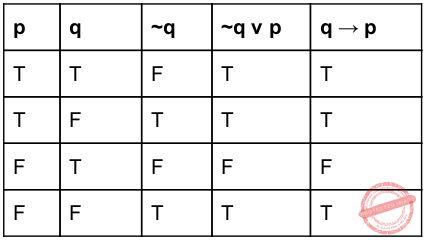Question 3
The number of edges in a complete graph with N vertices is equal to :
 A N (N−1) B 2N−1 C N−1 D N(N−1)/2
Engineering-Mathematics       Graph-Theory
Question 3 Explanation:
N =5Question 4
Which of the following is not true ?
 A Not given any option B A – B = A ∩~B C Not given any option D Not given any option
Engineering-Mathematics       Set-Theory
Question 4 Explanation:
A−B= A−(A∩B)
= A-(A∩B)
= A∩(A∩B)’
= A∩(A’ ∩ B’)
= (A∩A’)U(A∩B’)
= U(A∩B’)
= ​ ∅ U (​ A ∩ B’)
= A ∩ B’
 Question 5
If (a​ 2​ −b​ 2​ ) is a prime number where a and b ε N, then :
 A (a​ 2​ −b​ 2​ )​ =3 B (a​ 2​ −b​ 2​ )​ =a−b C (a​ 2​ −b​ 2​ )​ =a+b D (a​ 2​ −b​ 2​ )​ =5
Engineering-Mathematics       Set-Theory
Question 5 Explanation:
→ For any given numbers a and b which belongs to natural numbers , the options (A) and (D) are false
→ The set of natural numbers, denoted N, can be defined in the following ways: N = {0, 1, 2, 3, ...}
→ A prime number (or a prime) is a natural number greater than 1 that cannot be formed by multiplying two smaller natural numbers.
→ ‘a-b’ may be gives the non negative values which is not prime number but the a+b may give the prime value.
 Question 6
The hexadecimal equivalent of (10111)​ 2​ ×(1110)​ 2 is :
 A 150 B 241 C 142 D 101011110
Digital-Logic-Design       Number-Systems
Question 6 Explanation:
Step-1: First convert binary number into decimal number
(10111)​ 2​ = (23)​ 10
(1110)​ 2​ = (14)​ 10
Step-2: Perform multiplication 23*14=(322)​ 10
Step-3: Convert (322)​ 10​ into hexadecimal number (322)​ 10​ = (142)​ 16
 Question 7
An example of a self complementing code is :
 A 8421 code B Gray code C Excess-3 code D 7421 code
Digital-Logic-Design       Number-Systems
Question 7 Explanation:
→ Excess-3 code is also called Self-Complementing Code. Because 1’s complement of excess-3 number is equivalent to 9’s complement of corresponding decimal digit.
→ In excess-3 code, each of the 4-bit number represents decimal digit which is 3 less than actual decimal digit. So the bits have no fixed weight.
→ Excess-3 code is ​ neither​ CRC ​ nor​ Algebraic Code which are used for error detection and/or correction.
 Question 8
A sum of products expression can be implemented with __________ logic gates.
 A AND − OR B NAND − OR C AND − NOT D OR − AND
Digital-Logic-Design       Logic-Gates
Question 8 Explanation:
A sum of products expression can be implemented with AND-OR logic gates.
Ex: (AB)+(BC)+(CD)
 Question 9
The characteristic equation of the D flip-flop is :
 A Not option given B Q=D C Q=1 D Q=0 E Q​ t+1​ =D
Digital-Logic-Design       Sequential-Circuits
Question 9 Explanation:
D flip flop characteristic equation truth table: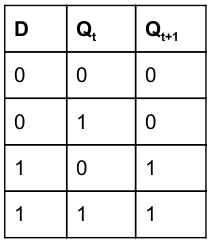Q​ t+1​ = DQ' + DQ
Q​ t+1​ =D
 Question 10
Which of the following logic is the fastest ?
 A RTL B ECL C HTL D HCL
Question 10 Explanation:
Emitter Coupled Logic (ECL)
The storage time is eliminated as the transistors are used in difference amplifier mode and are never driven into saturation.
1. Fastest among all logic families
2. Lowest propagation delay.
Resistor Transistor Logic (RTL)
Sometimes also transistor–resistor logic (TRL) is a class of digital circuits built using resistors as the input network and bipolar junction transistors (BJTs) as switching devices. RTL is the earliest class of transistorized digital logic circuit used; other classes include diode–transistor logic (DTL) and transistor–transistor logic (TTL).
 Question 11
When a function is recursively called, all automatic variables :
 A are initialized during each execution of the function B are retained from the last execution C are maintained in a stack D are ignored
Programming       Functions
Question 11 Explanation:
→ When a function is recursively called, all automatic variables are initialized during each execution of the function. → Automatic local variables primarily applies to recursive lexically-scoped languages. An automatic variable is a local variable which is allocated and deallocated automatically when program flow enters and leaves the variable's scope. The scope is the lexical context, particularly the function or block in which a variable is defined.
 Question 12
Enumeration variables can be used in :
 A search statement like an integer variable B break statement C preprocessor commands D function statement
Programming       Data-Type
Question 12 Explanation:
→ Enumeration variables can be used in search statement like an integer variable.
→ The enumerator names are usually identifiers that behave as constants in the language. An enumerated type can be seen as a degenerate tagged union of unit type. A variable that has been declared as having an enumerated type can be assigned any of the enumerators as a value.
→ In ‘C’ language exposes the integer representation of enumeration values directly to the programmer. Integers and enum values can be mixed freely, and all arithmetic operations on enum values are permitted. It is even possible for an enum variable to hold an integer that does not represent any of the enumeration values.
 Question 13
int arr[ ]={1, 2, 3, 4}
int count;
incr( )
{
return ++count;
}
main( )
{
arr[count++]=incr( );
printf(“arr[count]=%d\n”, arr[count]);
}
The value printed by the above program is ​ :
 A 1 B 2 C 3 D 4
Programming       Arrays
Question 13 Explanation:
Step-1: Before printing the arr[count] value, the variable “count” is incremented twice then count =2
Step-2: arr value is 3 , So, it will print the value “3”.
Method-2:
→ The function incr() is executed as it is first statement.
→ Count is global variable whose initial value is 0 ,the function definition increments the count value and returns value “1” to the main function.
→ Again the main function, the expression arr[count++] , here count variable operation is post increment so arr will store the value “1”.
→ After that statement , count value becomes “2” as it is incremented in the previous statement (total two increments).
→ Before printing the arr[count] value, the variable “count” is incremented twice then count =2
→ arr value is 3 , so it will print the value “3”.
 Question 14
When one-dimensional character array of unspecified length is assigned an initial value :
 A an arbitrary character is automatically added to the end of the string B ‘0’ is added to the end of the string C length of the string is added to the end of the string D ‘end’ is added to the end of the string
Programming       Arrays
Question 14 Explanation:
→ String literals are stored in ‘C’ as an array of chars, terminated by a null byte. A null byte is a char having a value of exactly zero, noted as '\0'.
→ When one-dimensional character array of unspecified length is assigned an initial value ‘0’ is added to the end of the string.
 Question 15
The declaration “unsigned u” indicates :
 A u is an unsigned character B u is an unsigned integer C u is a character D u is a string
Programming       Data-Type
Question 15 Explanation:
The declaration “unsigned u” indicates u is an unsigned integer.
 Question 16
Which possibility among the following is invalid in case of a Data Flow Diagram ?
 A A process having in-bound data flows more than outbound data flows B A data flow between two processes C A data flow between two data stores D A data store having more than one in-bound data flows
Question 16 Explanation:
DFD Rules:
1.Each process should have at least one input and an output.
2.Each data store should have at least one data flow in and one data flow out.
3.Data stored in a system must go through a process.
4.All processes in a DFD go to another process or a data store.
 Question 17
In DBMS, deferred update means :
 A All the updates are done first but the entries are made in the log file later B All the log files entries are made first but the actual updates are done later C Every update is done first followed by a writing on the log file D Changes in the views are deferred till a query asks for a view
Database-Management-System       ER-Model
Question 17 Explanation:
→ In deferred update techniques updates are stored to a local storage instead of storing onto the disk. In this technique, a updates is made first later when the transaction gets committed all entries are made in the log file(which is stored into disk) later.
→ If a transaction fails before reaching its commit point, it will not have changed the database in any way so UNDO is not needed. It may be necessary to REDO the effect of the operations that are recorded in the local transaction workspace, because their effect may not yet have been written in the database. Hence, a deferred update is also known as the No-undo/redo algorithm.
 Question 18
Which statement is false regarding data independence ?
 A Hierarchical data model suffers from data independence B Network model suffers from data independence C Relational model suffers only from logical data independence D Relational model suffers only from physical data independence
Database-Management-System       Relational-databases
Question 18 Explanation:
TRUE: Hierarchical data model suffers from data independence
TRUE: Network model suffers from data independence
FLASE: Relational model suffers only from logical data independence
TRUE: Relational model suffers only from physical data independence
 Question 19
Which of the following tools is not required during system analysis phase of system development life cycle ?
 A Case tool B RAD tool C Reverse engineering D None of these
Software-Engineering       Software-Development
Question 19 Explanation:
→ ​ Reverse engineering tools​ are using debuggers. The debuggers are either user mode or Kernel mode.
→ ​ Computer aided software engineering​ (CASE) is the domain of software tools used to design and implement applications. CASE tools are similar to and were partly inspired by computer aided design (CAD) tools used for designing hardware products. CASE tools are used for developing high-quality, defect-free, and maintainable software.
→ ​ Rapid Application Development​ refers to a software development approach which puts less emphasis on planning and even design specifications, and more on rapid development and quick feedback. Prototypes are rapidly delivered to users to ensure they’re directly involved in feedback and development. There are also tools for collecting data, designing personal workflows, and setting up unique rules for each new application.
 Question 20
Two phase protocol in a database management system is :
 A a concurrency mechanism that is not deadlock free B a recovery protocol used for restoring a database after a crash C Any update to the system log done in 2-phases D not effective in Database
Database-Management-System       Transactions
Question 20 Explanation:
The two phase protocol in DBMS refers to a protocol in which locking and unlocking of shared data includes two phases:
1. Growing Phase: In this locks are acquired according to the need as the transaction proceeds.
2. Shrinking Phase: In this release locks as transaction starts reaching to completion.
A transaction can obtain locks but can't release locks in growing phase. Similarly a transaction can release locks but can't obtain lock in shrinking phase.
There are various types of two phase locking mechanism which can provide a conflict serializable schedule but can't provide a schedule which is deadlock free and can be implemented practically.
 Question 21
Which algorithm has same average, worst case and best case time ?
 A Binary search B Maximum of n number C Quick sort D Fibonacci search
Algorithms       Time-Complexity
Question 21 Explanation: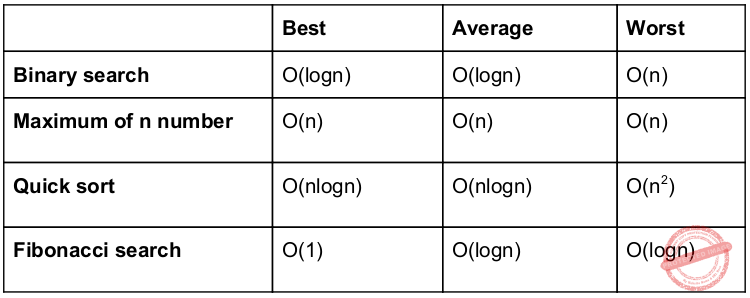Question 22
Binary search tree is an example of :
 A Divide and conquer technique B Greedy algorithm C Back tracking D Dynamic Programming
Data-Structures       Binary-Trees
Question 22 Explanation:
Binary search tree is an example of divide and conquer technique.
Procedure:
1. Divide: select lower or upper half
2. Conquer: search selected half
3. Combine: None
Recurrence relation​ : T(n)=T(n/2)+Θ(1)
Using masters theorem,
a=1, b=2, k=0,p=0
T(n)=Θ(logn)
 Question 23
What is the time required to insert an element in a stack with linked implementation ?
 A O (log​ 2​ n) B O (n) C O (n log​ 2​ n) D O (1)
Data-Structures       Queues-and-Stacks
Question 23 Explanation:
Average case in linked list: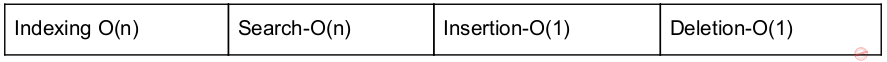Worst case in linked list:Question 24
The equivalent postfix expression for d/(e +f) +b*c
 A defbc/++* B def+/bc+* C def+/bc *+ D None of these
Data-Structures       Queues-and-Stacks
Question 24 Explanation: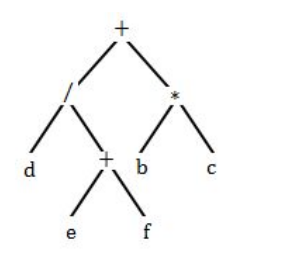Postfix expression: d ef + / bc * +
 Question 25
Which one of the following is a physical data structure ?
 A Array B Linked lists C Stacks D Tables
Data-Structures       Arrays
Question 25 Explanation:
→ An array is a number of elements in a specific order, typically all of the same type (depending on the language, individual elements may either all be forced to be the same type, or may be of almost any type).
→ Elements are accessed using an integer index to specify which element is required. Typical implementations allocate contiguous memory words for the elements of arrays (but this is not always a necessity). Arrays may be fixed-length or resizable.
Note: Arrays are directly stored in a memory location. Linked list, Stacks and table(using array) are used logical memory rather than physical memory.
 Question 26
How many DS1 signals are transported on a DS3 signal ?
 A 24 B 672 C 14 D 28
Question 26 Explanation:
→ A Digital Signal 3(DS3) is a digital signal level 3 T-carrier. It may also be referred to as a T3 line.
1.The data rate for this type of signal is 44.736 Mbit/s (45 Mb). 2.DS3 uses 75 ohm coaxial cable and BNC connectors. 3.This level of carrier can transport ​ 28​ ​ DS1​ level signals within its payload. 4.This level of carrier can transport ​ 672 DS0​ level channels within its payload.
 Question 27
A 10 BASE-2 network is limited to :
 A 20 bytes per data field B 30 stations per segment C 40 segments D 50 feet of cable
Question 27 Explanation:
→ A 100BASE-2 coaxial cables have a maximum length of 185 metres (607 ft).
→ The maximum practical number of nodes that can be connected to a 10BASE2 segment is limited to ​ 30​ with a minimum distance of 50 cm between devices.
→ In a 10BASE2 network, each stretch of cable is connected to the transceiver (which is usually built into the network adaptor) using a BNC T-connector, with one stretch connected to each female connector of the T. The T-connector must be plugged directly into the network adaptor with no cable in between.
 Question 28
The network 198:78:41:0 is a :
 A Class A Network B Class B Network C Class C Network D Class D Network
Question 28 Explanation:Question 29
The subnet mask 255.255.255.192
 A Extends the network portion to 16 bits B Extends the network portion to 26 bits C Extends the network portion to 36 bits D Has no effect on the network portion of an IP address
Question 29 Explanation:
Default subnet mask for Class-C is 255.255.255.192
(192)​ 10​ = (11000000)​ 2
Since, 192 is written as 11000000, it has 2 sub-nets and remaining all hosts.
So, for first three octets, 24 bits are fixed and for last octet 2 bits are fixed, i.e. 24 + 2 = 26 bits
 Question 30
The LAPB frame structure and the frame structure of SDLC are :
 A Opposite B Identical C Reversed D Non-identical
Question 30 Explanation:
Link Access Procedure, Balanced (LAPB) implements the data link layer as defined in the X.25 protocol suite. LAPB is a bit-oriented protocol derived from HDLC that ensures that frames are error free and in the correct sequence.
Frame format:*** The LAPB frame structure and the frame structure of SDLC are identical.
 Question 31
 A cannot be performed before relocation B cannot be performed after relocation C can be performed both before and after relocation D is not required if relocation is performed
Question 31 Explanation:
Linking cannot be performed after relocation.
Program execution steps:Question 32
Which of the following is the most general phase-structured grammar ?
 A Regular B Context-sensitive C Context free D Syntax tree
Theory-of-Computation       Languages-and-Grammars
Question 32 Explanation:
→ Phrase structure grammars are also known as constituency grammars. The defining trait of phrase structure grammars is thus their adherence to the constituency relation, as opposed to the dependency relation of dependency grammars.
→ Most general phase structured grammar is context sensitive grammar.
 Question 33
A compiler for a high level language that runs on one machine and produces code for a different machine is called :
 A Optimizing B One pass compiler C Cross compiler D Multipass compiler
Compiler-Design       Compilers
Question 33 Explanation:
→ Incremental compiler: The compiler which compiles only the changed lines from the source code and update the object code
→ Threaded code compiler: The compiler which simply replace a string by an appropriate binary code.
→ Cross compiler: The compiler used to compile a source code for different kinds platform.
**One pass assembler and two pass assemblers are available.
 Question 34
The ‘K’ in LR(R) cannot be :
 A 0 B 1 C 2 D None of these
Compiler-Design       Parsers
Question 34 Explanation:
→ The name LR is often followed by a numeric qualifier, as in LR(1) or sometimes LR(k). To avoid backtracking or guessing, the LR parser is allowed to peek ahead at k lookahead input symbols before deciding how to parse earlier symbols.
→ Typically k is 1 and is not mentioned. The name LR is often preceded by other qualifiers, as in SLR and LALR. The LR(k) condition for a grammar was suggested by Knuth to stand for "translatable from left to right with bound k."
 Question 35
Peer-hole optimization is a form of :
 A loop optimization B local optimization C constant folding D data flow analysis
Compiler-Design       Code-Optimization
Question 35 Explanation:
→ Peephole optimization is a kind of optimization performed over a very small set of instructions in a segment of generated code. The set is called a "peephole" or a "window". It works by recognizing sets of instructions that can be replaced by shorter or faster sets of instructions.
Common techniques:
Null sequences
Combine operations
Algebraic laws
Special case instructions
Constant folding
 Question 36
An operating system is :
 A Collection of hardware components B Collection of input-output devices C Collection of software routines D All the above
Operating-Systems       I/O-Management
Question 36 Explanation:
An operating system is collection of software routines. Hardware and I/O devices are different to operating system.
 Question 37
____________ is one of preemptive scheduling algorithm.
 A Shortest-Job-first B Round-robin C Priority based D Shortest-Job-next
Operating-Systems       Process-Scheduling
Question 37 Explanation:
→ SJF scheduling algorithm is either preemptive and Nonpreemptive.
→ Round robin is purely Nonpreemptive.
→ Priority based scheduling also preemptive and Nonpreemptive.
→ Shortest-Job-next scheduling algorithm is either preemptive and Nonpreemptive.
 Question 38
A software to create a Job Queue is called ____________ .
 A Linkage editor B Interpreter C Driver D Spooler
Question 38 Explanation:
→ Spooling is a process in which data is temporarily held to be used and executed by a device, program or the system. Data is sent to and stored in memory or other volatile storage until the program or computer requests it for execution.
→ A software to create a Job Queue is called spooler. The spooler maintains an orderly sequence of jobs for the peripheral and feeds it data at its own rate.
Ex: Printer.
 Question 39
A permanent database of a general model of compiler is ____________ .
 A Identifier table B Page map table C Literal table D Terminal table
Compiler-Design       Compilers
Question 39 Explanation:
A permanent database of a general model of compiler is terminal table. A permanent database that has entry for each terminal symbols such as arithmetic operators, keywords, punctuation characters such as ‘;’, ‘,’etc Fields: Name of the symbol.
 Question 40
Loading operating system from secondary memory to primary memory is called ____________ .
 A Compiling B Booting C Refreshing D Reassembling
Operating-Systems       Memory-Management
Question 40 Explanation:
Loading operating system from secondary memory to primary memory is called booting.
 Question 41
Software Cost Performance index (CPI) is given by :
[Where : BCWP stands for Budgeted Cost of Work Performed.
BCWS stands for Budget Cost of Work Scheduled
ACWP stands for Actual Cost of Work Performed
 A BCW P/ ACW P B No option given C BCWP−ACWP D BCWP−BCWS
Software-Engineering       Software-configuration-management
Question 41 Explanation:
The Cost Performance Index can be determined by dividing the earned value by actual cost.
Cost Performance Index = BCWP stands for Budgeted Cost of Work Performed / ACWP stands for Actual Cost of Work Performed
CPI = BCWP/ACWP
 Question 42
Software Risk estimation involves following two tasks :
 A risk magnitude and risk impact B risk probability and risk impact C risk maintenance and risk impact D risk development and risk impact
Software-Engineering       Software-configuration-management
Question 42 Explanation:
Software Risk estimation involves risk probability and risk impact.
 Question 43
In a object oriented software design, ‘Inheritance’ is a kind of __________ .
 A relationship B module C testing D optimization
Programming-in-c++       Properties
Question 43 Explanation:
→ Inheritance refers to a particular relationship that two or more classes can share.
→ Polymorphism refers to a particular feature of classes that define an inheritance relationship.
 Question 44
Reliability of software is directly dependent on :
 A quality of the design B number of errors present C software engineer’s experience D user requirement
Software-Engineering       Software-Reliabiliy
Question 44 Explanation:
→ Reliability of software is dependent on number of errors present in software.
→ Software Reliability is the probability of failure-free software operation for a specified period of time in a specified environment.
 Question 45
‘Abstraction’ is ____________ step of Attribute in a software design.
 A First B Final C Last D Middle
Software-Engineering       Software-design
Question 45 Explanation:
‘Abstraction’ is first step of Attribute in a software design. The process of removing physical, spatial, or temporal details or attributes in the study of objects or systems in order to more closely attend to other details of interest.
 Question 46
The frequency band allocated for the downlink in GSM is :
 A 960 - 985 MHz B 935 - 960 MHz C 920 - 945 MHz D 930 - 955 MHz
Question 46 Explanation:
There are 2 popular GSM methods.
1. GSM-900
2. GSM-1800
GSM-900 uses 890 - 915 MHz to send information from the Mobile Station to the Base
Transceiver Station (uplink) and 935 - 960 MHz for the other direction (downlink), providing 124 RF channels (channel numbers 1 to 124) spaced at 200 kHz. Duplex spacing of 45 MHz is used.
 Question 47
Which of the following is an EDI standard ?
 A ANSI X.15 B ANSI X.14 C ANSI X.13 D ANSI X.12
Question 47 Explanation:
ANSI X.12: Chartered by ANSI (the American National Standards Institute) the Accredited Standards Committee (ASC) X12 develops, maintains, interprets, publishes and promotes the proper use of Electronic Data Interchange Standards. The ASC X12 standard has become the de-facto standard of choice for US-based EDI operations.
 Question 48
An INT file in Windows 95 is :
 A a program file B a message file C a text file D link file
Question 48 Explanation:
An INT file in Windows 95 is a text file. The INI file format is an informal standard for configuration files for some computing platforms or software. INI files are simple text files with a basic structure composed of sections, properties, and values.
 Question 49
Link analysis operation in data mining uses ___________ technique.
 A Classification B Association discovery C Visualisation D Neural clustering
Question 49 Explanation:
→ Link analysis operation in data mining uses Association discovery technique.
→ This rule-based approach also generates new rules as it analyzes more data. The ultimate goal, assuming a large enough dataset, is to help a machine mimic the human brain’s feature extraction and abstract association capabilities from new uncategorized data.
 Question 50
The maximum size of SMS in IS-95 is ______ octets.
 A 120 B 95 C 128 D 64
Question 50 Explanation:
The maximum size of SMS in IS-95 is 120 octets.
There are 50 questions to complete.
PHP Code Snippets Powered By : XYZScripts.com